Function Repository Resource:

# FindExtraordinaryLines

Return the lines passing through three or more points, given a set of points

Contributed by: Ed Pegg Jr
 ResourceFunction["FindExtraordinaryLines"][pts] returns indices for sets of three or more points in pts lying on the same line.

## Details

For a finite set of points, a line going through only two points is called an ordinary line.

## Examples

### Basic Examples (2)

Here are 11 points that make 15 lines of three points each:

 In:=Out=A graphic showing the points and lines:

 In:=Out=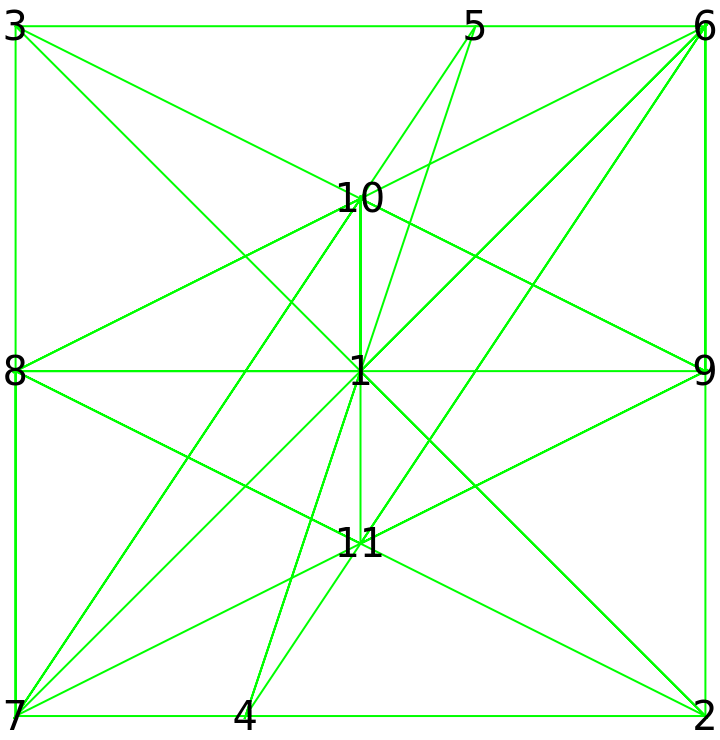### Scope (4)

Find all the lines of 3 or more points in a 3×3×3 lattice:

 In:=Out=In:=Out=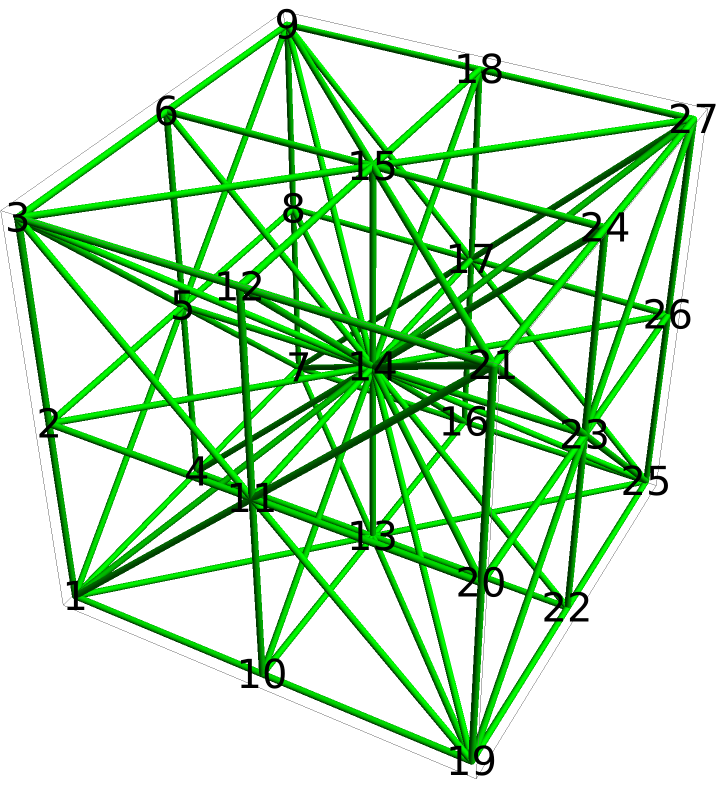Here is a set of 20 lattice points where all lines are ordinary:

 In:=Out=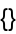In:=Out=Most sets of 20 points from this lattice will have many extraordinary lines:

 In:=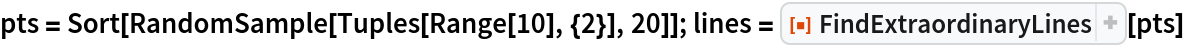Out=In:=Out=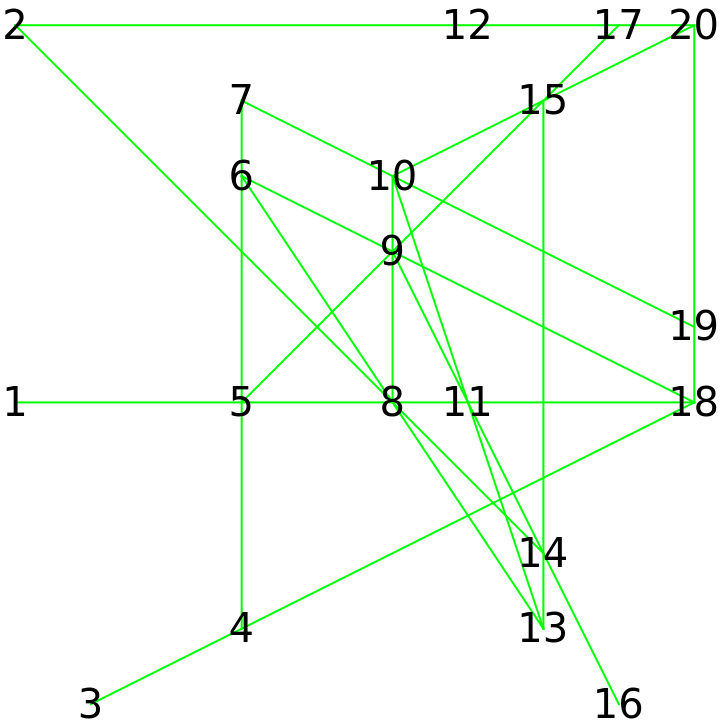Find lines going through powers of a complex solution for x3=x+1:

 In:=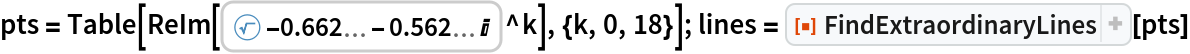Out=In:=Out=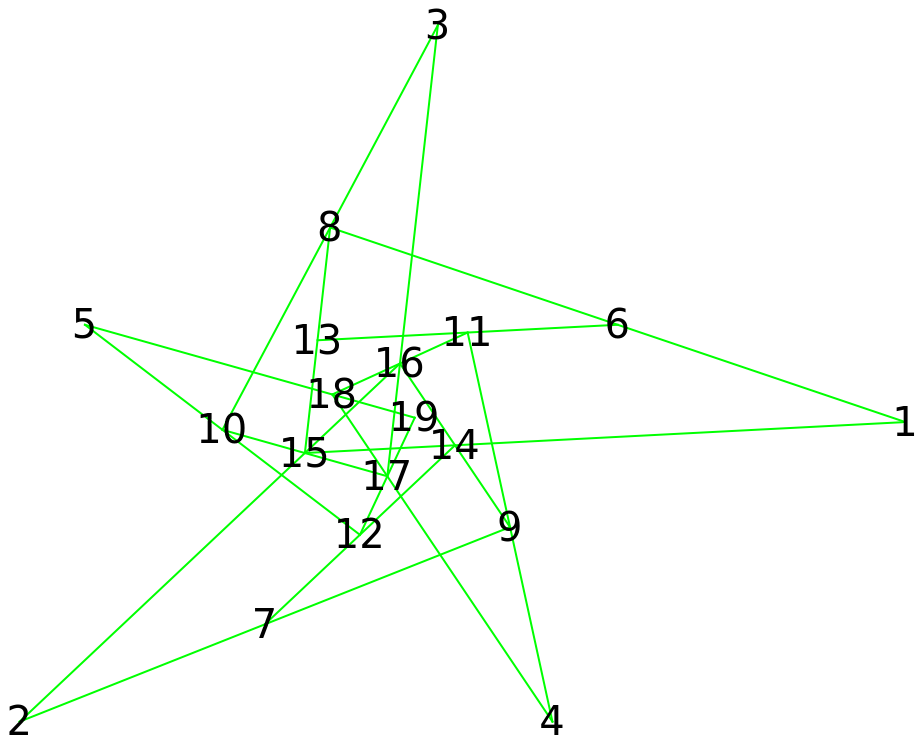## Requirements

Wolfram Language 11.3 (March 2018) or above

## Version History

• 2.0.1 – 22 November 2021